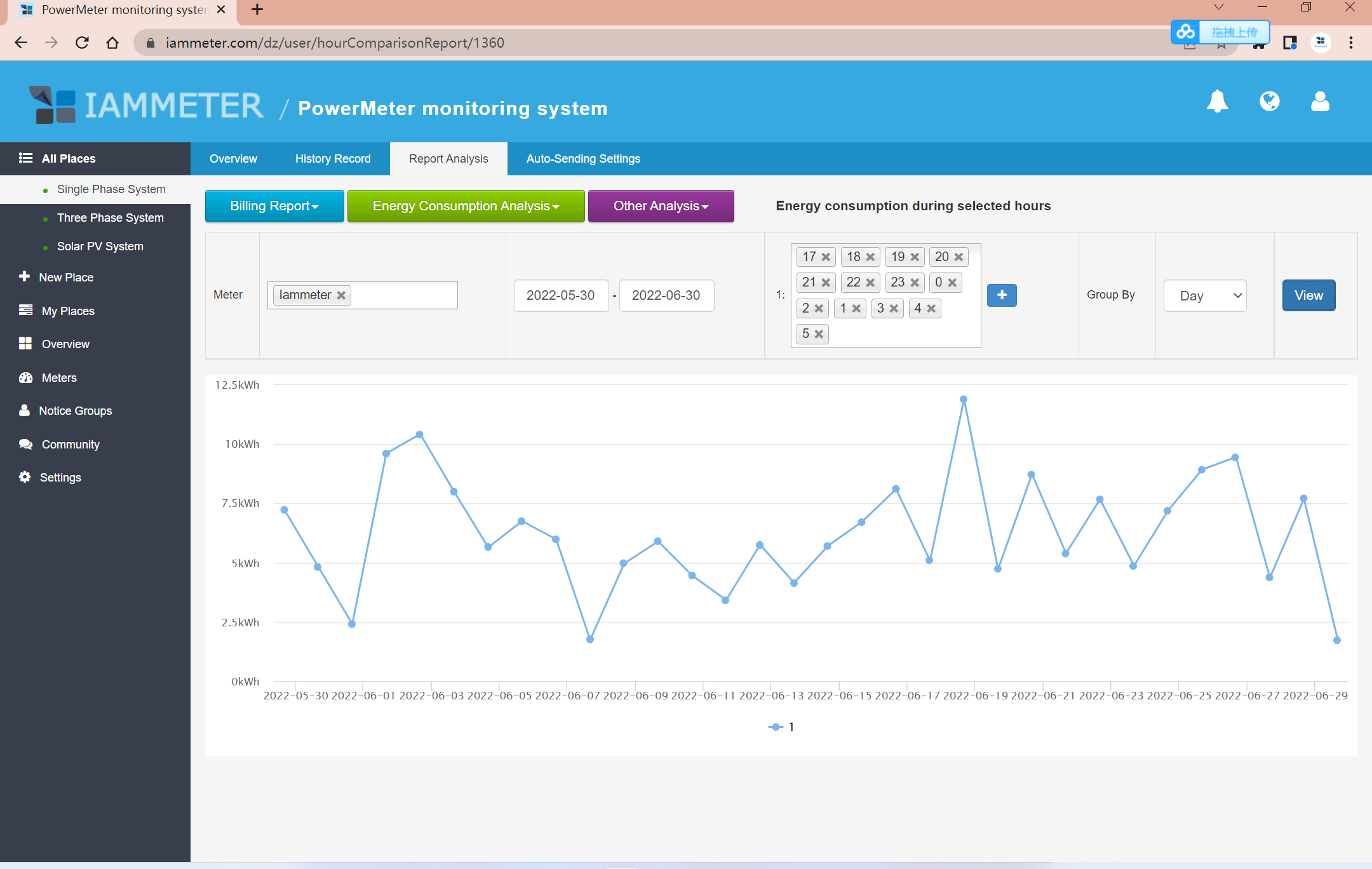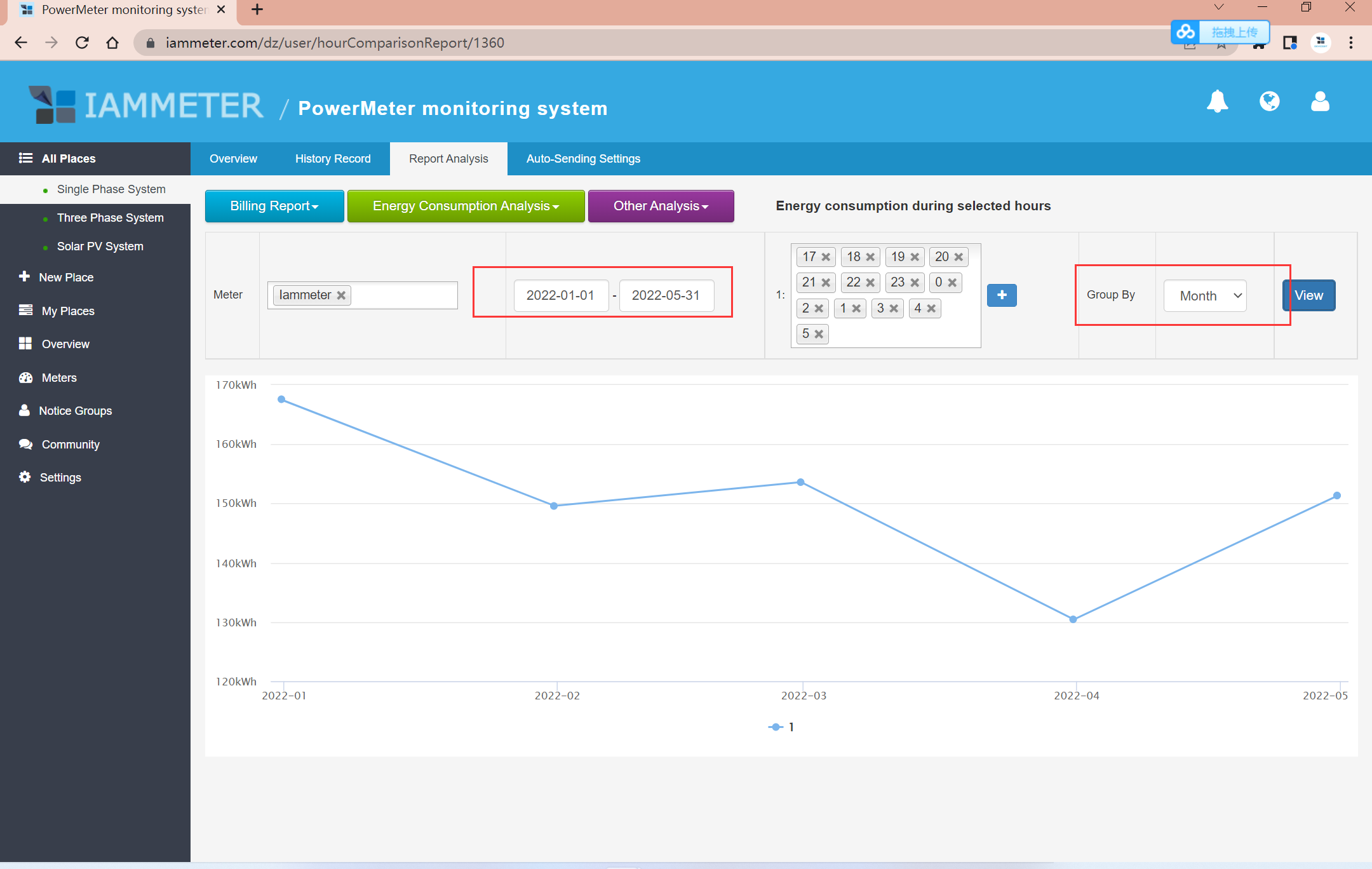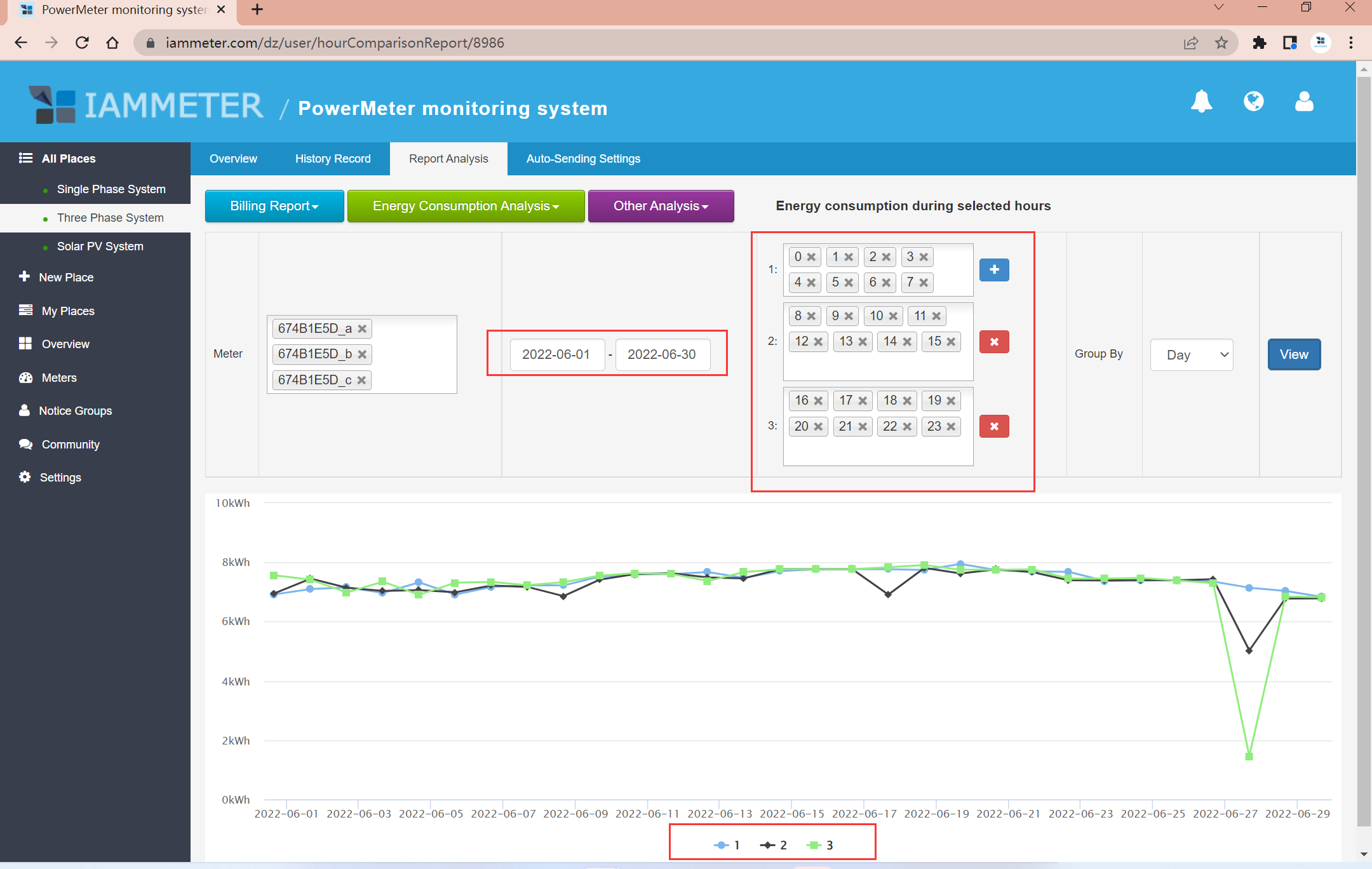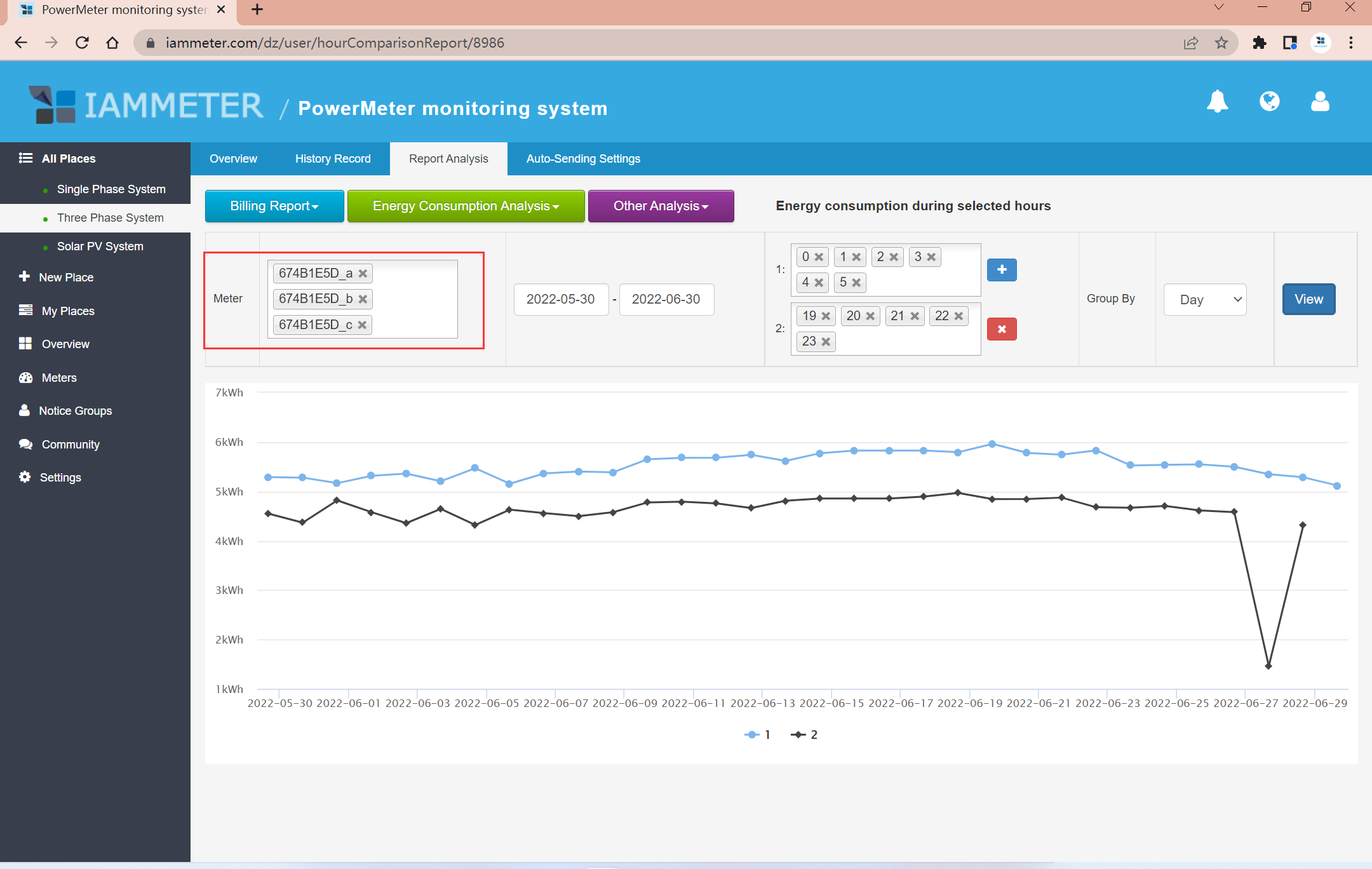### [New feature] Analyze the kilowatt-hours (kWh) in selected hours (Per day/month )

Report: Analyze the kilowatt-hours (kWh) in selected hours

How does it work

Analyze the kilowatt-hours (kWh) in selected hours per day(month)

Compare the kilowatt-hours (kWh) data in the different selected hours

Sum more than one energy meter together and analyze the kwh in selected hours

Summary of the new features of IAMMETER recently

This new report function comes from one suggestion of our customers in this URL Please kindly share your suggestion about the WiFi energy meter of IAMMETER

Hi, I am trying to calculate the size of the battery backup that I need for my house. In order to do so it would be helpful if there is a way to calculate the total (grid-) consumption during certain hours, as example from 17.00 to 0.700 when the sun is low or under. This would be needed not only per day but also per month or year, to see the general usage and if there are extremes in usage.

# How does it work

## Analyze the kilowatt-hours (kWh) in selected hours per day(month)

You can select the hours you want to analyze. The chart displays is the kwh that consumed or yielded in your selected hours every day.Group by: Month/Day

This means analyzing the kwh data in selected hours, in the unit of the month/day.

Ex: In below chart, each point in the chart is the sum of kwh in the selected hours in a month.## Compare the kilowatt-hours (kWh) data in the different selected hours

This report also supports comparing the kwh data from more selected hours.

This function is very useful in the following scenarios. The energy meter is been installed in a factory where there are three work times for the different working groups(0:00-8:00,8:00-16:00 and 16:00-24:00). You want to get the kilowatt-hours (kWh) for each group and analyze the work efficiency.

Then you can configure this report like below pics## Sum more than one energy meter together and analyze the the kwh in selected hours

please note, that if you select more than one meter in the meter field. it means you want to analyze the sum of the meters you selected. Ex: if you select only one phase in meter, the chart will output the kwh in one phase in selected hours. If you select all three phases, the chart will output all thee sum kwh (total kilowatt-hours) data in selected hours.

So if you want to analyze the net energy metering mode(NEM) result in selected hours, please select the NEM meter (the fourth) in the meter`s field. About Net energy metering mode(NEM), please refer to https://www.iammeter.com/docs/net-energy-meter# Summary of the new features of IAMMETER recently

All new features about IAMMETEW will be published in our official forum first. https://imeter.club/Monitor your solar pv system by Wi-Fi Energy meter

Integrate W-Fi Energy meter into Home Assistant and monitor your solar pv system in Home assistant

Three phase energy meter that support modbus RTU/TCP ,mqtt, net energy metering,NodeRed,Home Assistant,Openhab

Analyze the energy difference within the selected time interval from the power chart

Demand charge report in IAMMETER

Compare the kwh/period data of wifi energy meter.

Virtual Site